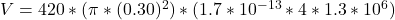## A flat coil of wire is used with an LC-tuned circuit as a receiving antenna. The coil has a radius of 0.30 m and consists of 420 turns. The

Question

A flat coil of wire is used with an LC-tuned circuit as a receiving antenna. The coil has a radius of 0.30 m and consists of 420 turns. The transmitted radio wave has a frequency of 1.3 MHz. The magnetic field of the wave is parallel to the normal of the coil and has a maximum value of 1.7 x 10-13 T. Using Faraday’s Law of electromagnetic induction and the fact that the magnetic field changes from zero to its maximum value in one-quarter of a wave period, find the magnitude of the average emf induced in the antenna in this time.

in progress 0
4 weeks 2021-08-31T14:50:59+00:00 1 Answers 0 views 0

The average  emf induce is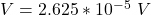Explanation:

From the question we are told that

The radius of the coil is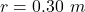The number of turns is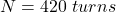The frequency of the transition radio wave is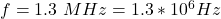The magnetic field is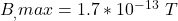The time taken for the magnetic field to go from zero to maximum is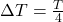The period of the transmitted radio wave is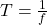So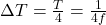The potential difference can be mathematically represented as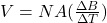Where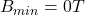substituting values